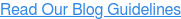### Blog: Subscribe NowFeatured Podcast

Listen Now

# Game Highlight: Visual Addition and Subtraction with ST Math

According to the 2017 National Assessment of Educational Progress (NAEP), only 40% of fourth graders are considered proficient in math -- a number that hasn’t changed since 2015. By eighth grade, only 34% of students are considered proficient, and the numbers don’t get better from there. So how can we make a difference?

Because true math understanding is a web of interconnected concepts (what we call schemas) rather than standalone facts, it’s crucial that students build rock-solid foundations from the beginning. If students don’t have a deep understanding of foundational concepts like addition and subtraction, we can’t expect them to master multiplication or tackle fractions. But if we use the right tools to encourage conceptual learning early on, we could see more students succeed into later grades.

What follows are two examples of ST Math® games that visually model addition and subtraction. The animated feedback and progression from visual to symbolic problem solving allows students to develop deep, conceptual understanding that carries over to more complex mathematics later on.

Like all ST Math games, the interface is devoid of any words, videos or elements that do not relate to the student’s hands-on experience of mathematical problem solving. The game objective is always the same: get JiJi the penguin across the screen.

This game is in a first grade addition module under “Addition and Subtraction Within Ten.” It begins with a concept that most students are already familiar with: counting. In the first levels, students count the floating blocks and select the correct number below. Notice how the blocks are in two groups on the left and right: the animations are setting the scene for a symbolic addition problem. Representing the sum with blocks on the ground also lays the foundation for using a number line which is introduced in a later objective.Even if students are already familiar and comfortable with counting, the animated response to each of their selections solidifies that understanding and models why their thinking worked. These first levels allow the students to get a feel for the game before scaling the difficulty and introducing mathematical symbols along with the visuals.

For those students who don’t catch on right away, the animated response, or informative feedback, shows exactly what went wrong. In this example of a more difficult level, the student chooses too many blocks because three are clearly left over:Each animation responds to the student’s action, showing feedback at the critical time where learning is actually taking place. Whether students select the wrong or the right answer, ST Math’s informative feedback visually models how the math works.

### Basic Fact Subtraction

The same game mechanics carry over to the next module on subtraction. In this game, the blocks are similarly grouped on the left and right to represent the order of an equation. The way that students select their answer remains consistent so they can smoothly build on their existing knowledge of addition. A notable difference is that the blocks on the ground are now grouped in fives. Teachers can encourage their students to compare each set of blocks to a full row and encourage fluency in adding and subtracting within 10 by using a benchmark of 5.In the next game, you can see that the all visual block equation has been partly replaced by a number. Once the student selects an answer in this level, the animation connects the number to the visual block representation from before.  Through all grades and subjects, ST Math transitions from a visual representation to a gradual inclusion of words, mathematical symbols and operations once students reach higher level games.  This scaffolding of the visual to symbolic, teaches students to problem solve in all settings, including equations and word problems### Rich Variety of Visual Models

No matter how well one visual model demonstrates addition and subtraction, students need to experience a variety of different situations for comprehensive conceptual understanding. The same ST Math objective that houses Select Box Addition and Basic Fact Subtraction, has three other visual models that represent the same functions:Try some of them for yourself by checking out the sample games on our website!

If you enjoyed this dive into ST Math, here are some easy links to more resources: